> 文档中心 > 【LeetCode】set集合+哈希表+快慢指针

# 【LeetCode】set集合+哈希表+快慢指针

LC热评 这就是我的真实想法（呜呜呜set集合本身的值是不重复的 ，无序的

# 349. 两个数组的交集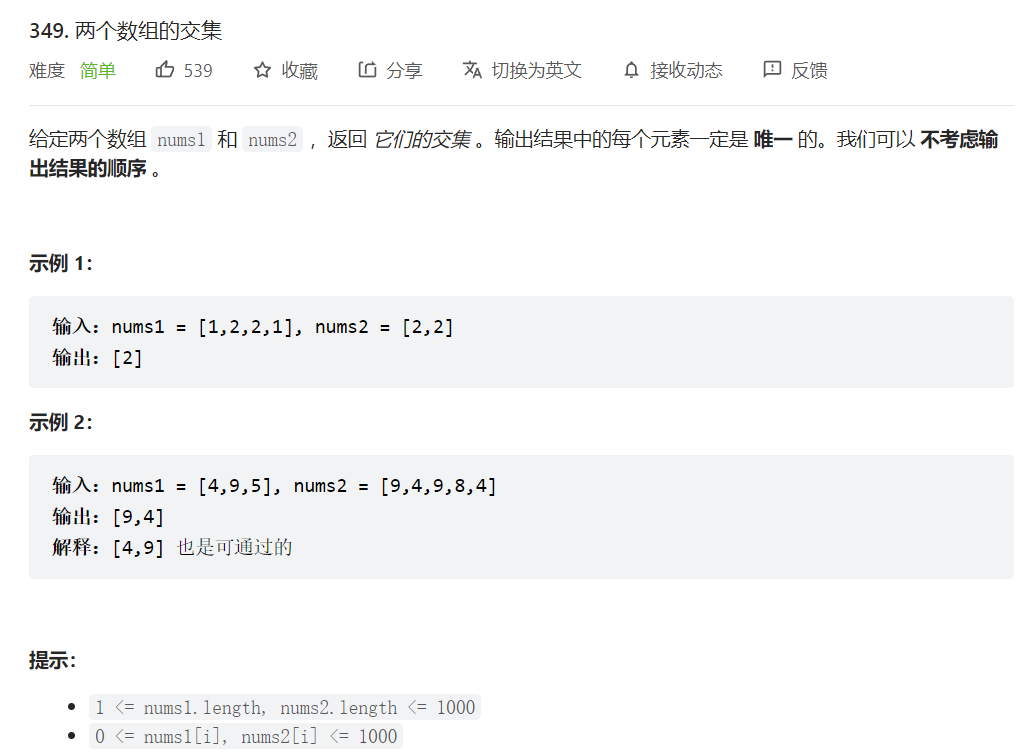## set的使用

1.创建set集合存储一个数组的值
2.如果set的值在另一个数组里存在，那就将该值存储在另一个set集合中
3.将set转化为数组即可

``class Solution {    public int[] intersection(int[] nums1, int[] nums2) { if(nums1 ==null || nums2 == null || nums2.length == 0 || nums1.length == 0) return new int;  Set<Integer>  set1 = new HashSet<>();  Set<Integer>  set2 = new HashSet<>();   for( int i : nums1){set1.add(i);   }   for(int i :nums2){if(set1.contains(i)){    set2.add(i);}   }   int[] sum = new int[set2.size()];   int index= 0;   for(int i : set2){sum[index++] = i;   }     return sum;    }}``

# 350. 两个数组的交集 II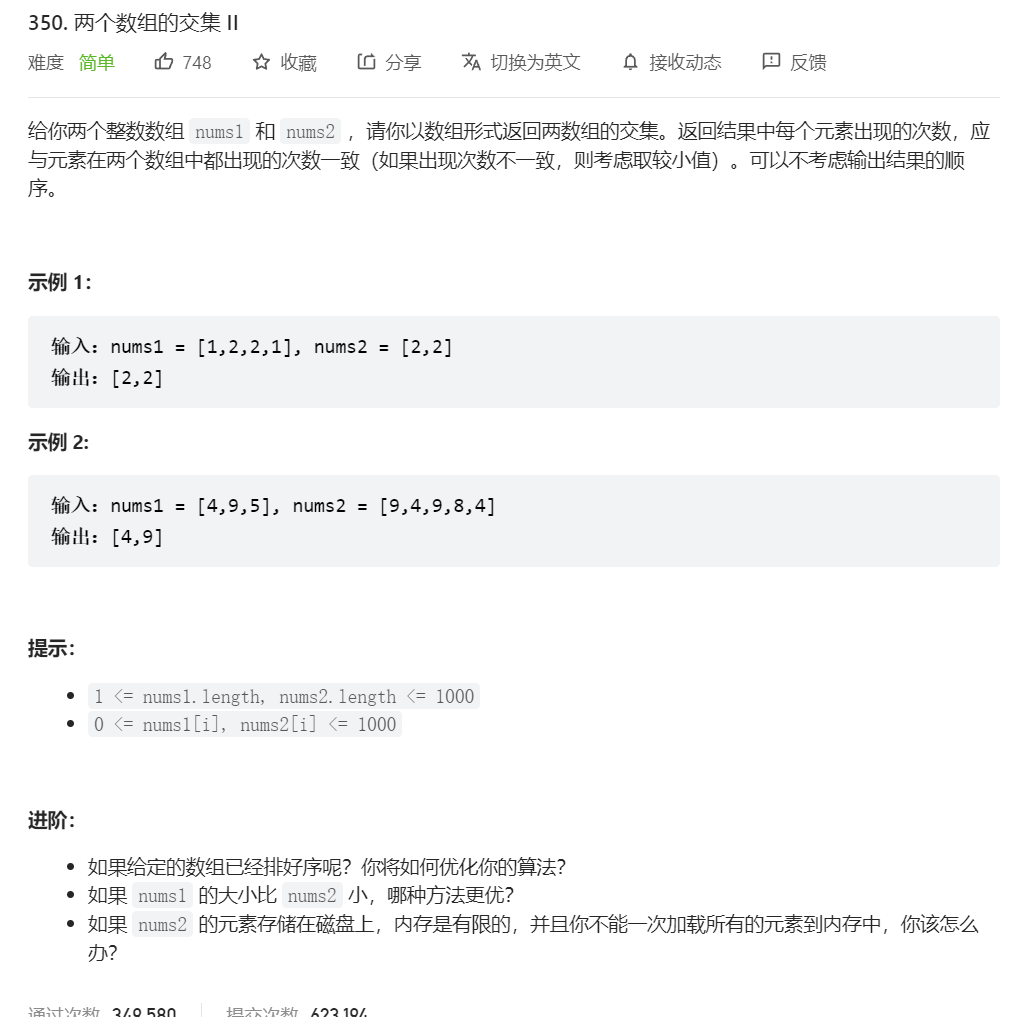## 方法一：哈希表

map 的key 为nums2的数字，value为nums2数字出现的次数

``class Solution {    public int[] intersect(int[] nums1, int[] nums2) { if(nums1.length > nums2.length){     return intersect(nums2,nums1); } Map<Integer,Integer> map  = new HashMap<Integer,Integer>(); for( int i :nums2){     int  num =map.getOrDefault(i,0);     map.put(i,num+1);  } int[] sum = new int[nums1.length]; int index  =0 ; for(int i : nums1){     int count = map.getOrDefault(i,0);     if(count > 0){  count--;  sum[index++] = i;  if(count >0){      map.put(i,count);  }else{      map.remove(i);  }     } } return Arrays.copyOfRange(sum,0,index);    }}``

## 第二种方法：排序 + 双指针

``class Solution {    public int[] intersect(int[] nums1, int[] nums2) { Arrays.sort(nums1); Arrays.sort(nums2); int [] sum = new int[Math.min(nums1.length,nums2.length)]; int index1 = 0,index2 = 0, index = 0; while(index1 <nums1.length && index2 <nums2.length){     if(nums1[index1] < nums2[index2]){  index1++;     }else if(nums1[index1] > nums2[index2]){  index2++;     }else{  index2++;  sum[index++] = nums1[index1++];  } } return Arrays.copyOfRange(sum,0,index);    }}``## 202. 快乐数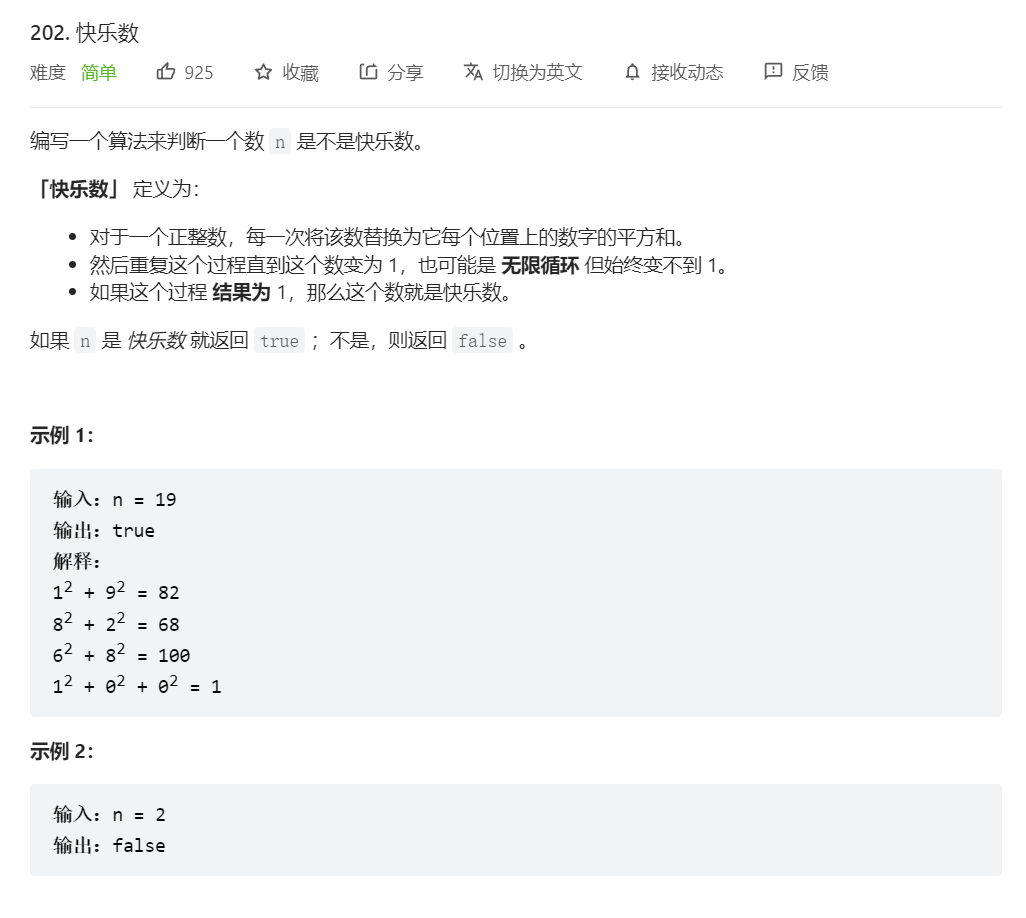## 哈希表做法

1.如果他是快乐数，那他就不会进入循环。2.当他不是快乐数时，他最终还是会进入循环。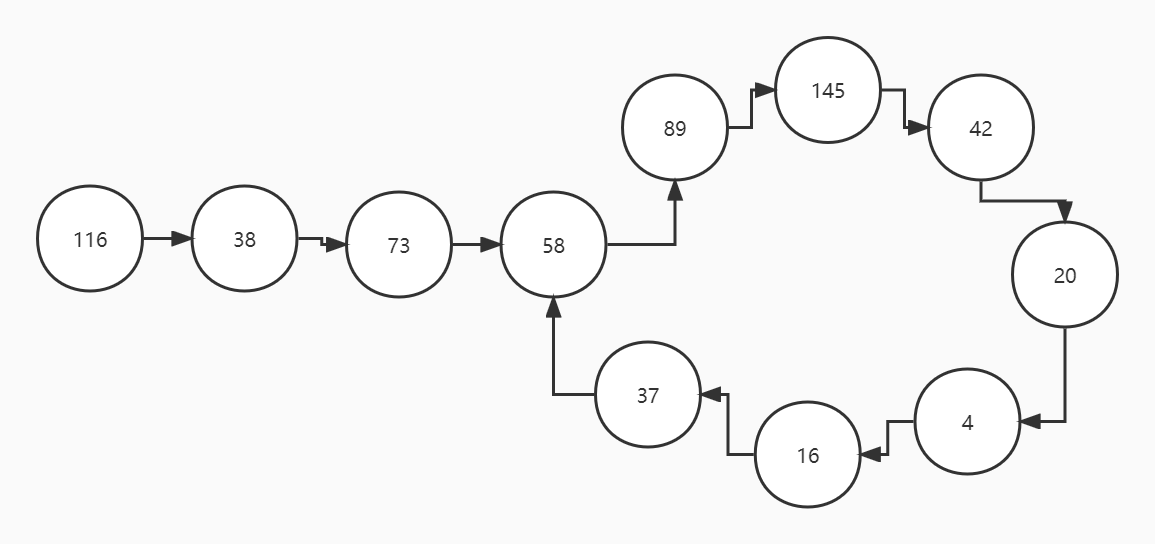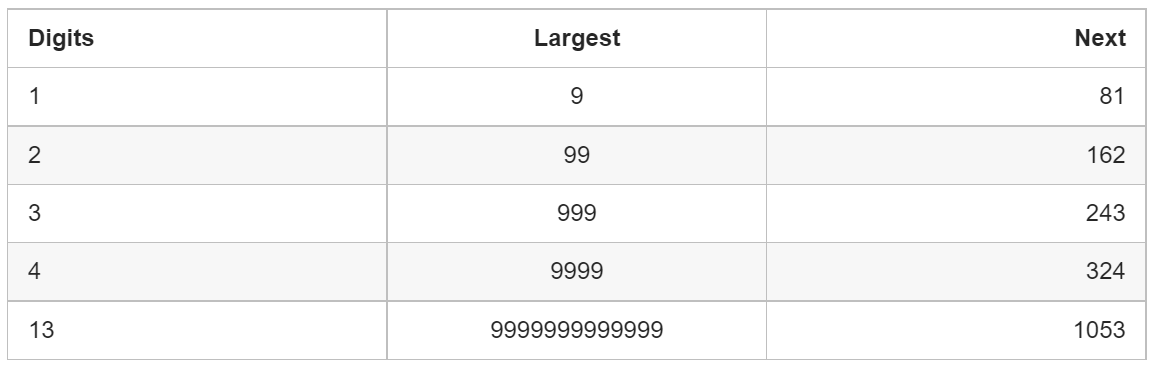1.给一个数字 nn，它的下一个数字是什么？
2.按照一系列的数字来判断我们是否进入了一个循环。

1.如果它不在哈希集合中，我们应该添加它。
2.如果它在哈希集合中，这意味着我们处于一个循环中，因此应该返回 `false`

``class Solution {    public boolean isHappy(int n) { Set<Integer> set = new HashSet<>(); while(n != 1 && !set.contains(n)){     set.add(n);     n = getNextNumber(n); } return n == 1;    }    private int getNextNumber( int n ){ int res = 0; while(n > 0){     int i = n % 10;     res += i*i;     n = n /10; } return res;    }}``

## 方法二：快慢指针

``class Solution {    public boolean isHappy(int n) {int fast = n , slow =n;do{    slow = getNextNumber(slow);    fast = getNextNumber(fast);    fast = getNextNumber(fast);}while(fast != slow);return fast ==1;    }    private int getNextNumber( int n ){ int res = 0; while(n > 0){     int i = n % 10;     res += i*i;     n = n /10; } return res;    }}``